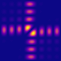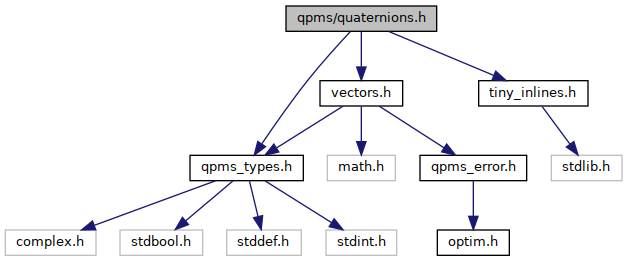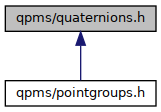QPMS Electromagnetic multiple scattering library and toolkit.
quaternions.h File Reference

Quaternions and Wigner matrices. More...

#include "qpms_types.h"
#include "vectors.h"
#include "tiny_inlines.h"
Include dependency graph for quaternions.h:This graph shows which files directly or indirectly include this file:Go to the source code of this file.

## Macros

#define QPMS_QUAT_ATOL   (1e-10)
Just some arbitrarily chosen "default" value for quaternion comparison tolerance.

## Typedefs

using q = qpms_quat_normalise(q)

## Functions

static qpms_quat_t qpms_quat_2c_from_4d (qpms_quat4d_t q)
Conversion from the 4*double to the 2*complex quaternion.

static qpms_quat4d_t qpms_quat_4d_from_2c (qpms_quat_t q)
Conversion from the 2*complex to the 4*double quaternion.

static qpms_quat_t qpms_quat_mult (qpms_quat_t p, qpms_quat_t q)
Quaternion multiplication. More...

static qpms_quat_t qpms_quat_add (qpms_quat_t p, qpms_quat_t q)

static qpms_quat_t qpms_quat_sub (qpms_quat_t p, qpms_quat_t q)
Quaternion substraction.

static qpms_quat_t qpms_quat_exp (const qpms_quat_t q)
Exponential function of a quaternion.

Quaternion scaling with a real static number qpms_quat_t qpms_quat_rscale (double s, qpms_quat_t q)

Quaternion static conjugation qpms_quat_t qpms_quat_conj (const qpms_quat_t q)

Quaternion static norm double qpms_quat_norm (const qpms_quat_t q)

Test approximate equality of static quaternions bool qpms_quat_isclose (const qpms_quat_t p, const qpms_quat_t q, double atol)

Standardises a quaternion to have the largest component static positive qpms_quat_t qpms_quat_standardise (qpms_quat_t p, double atol)

Test approximate equality of standardised so that f q f is considered equal to f \$q static f bool qpms_quat_isclose2 (const qpms_quat_t p, const qpms_quat_t q, double atol)

Norm of the quaternion imaginary (vector) part. static inline double qpms_quat_imnorm(const qpms_quat_t q)

Quaternion normalisation to unit static norm qpms_quat_t qpms_quat_normalise (qpms_quat_t q)

Logarithm of a static quaternion qpms_quat_t qpms_quat_log (const qpms_quat_t q)

Quaternion power to a real static exponent qpms_quat_t qpms_quat_pow (const qpms_quat_t q, const double exponent)

Quaternion static inversion qpms_quat_t qpms_quat_inv (const qpms_quat_t q)

Make a pure imaginary quaternion from a cartesian static vector qpms_quat_t qpms_quat_from_cart3 (const cart3_t c)

Make a cartesian vector from the imaginary part of a static quaternion cart3_t qpms_quat_to_cart3 (const qpms_quat_t q)

return qpms_quat_to_cart3 (qpms_quat_mult(q, qpms_quat_mult(vv, qc)))

Versor quaternion from rotation vector (norm of the vector is the rotation angle). static inline qpms_quat_t qpms_quat_from_rotvector(cart3_t v)

Wigner D matrix element from a rotator quaternion for integer a l complex double qpms_wignerD_elem (qpms_quat_t q, qpms_l_t l, qpms_m_t mp, qpms_m_t m)

A VSWF representation element of the O (3) group. complex double qpms_vswf_irot_elem_from_irot3(const qpms_irot3_t q

static int qpms_irot3_checkdet (const qpms_irot3_t p)

Improper rotation static multiplication qpms_irot3_t qpms_irot3_mult (const qpms_irot3_t p, const qpms_irot3_t q)

Improper rotation inverse static operation qpms_irot3_t qpms_irot3_inv (qpms_irot3_t p)

Improper rotation power f p n static f qpms_irot3_t qpms_irot3_pow (const qpms_irot3_t p, int n)

Test approximate equality of static irot3 bool qpms_irot3_isclose (const qpms_irot3_t p, const qpms_irot3_t q, double atol)

Apply an improper rotation onto a cartesian static vector cart3_t qpms_irot3_apply_cart3 (const qpms_irot3_t p, const cart3_t v)

versor representing rotation around z static axis qpms_quat_t qpms_quat_zrot_angle (double angle)

versor representing rotation f C_N k i e of angle f pi k N f around z static axis qpms_quat_t qpms_quat_zrot_Nk (double N, double k)

Rotation around z static axis qpms_irot3_t qpms_irot3_zrot_angle (double angle)

Rotation f C_N k i e of angle f pi k N f around z static axis qpms_irot3_t qpms_irot3_zrot_Nk (double N, double k)

## Variables

Quaternion real static unit const qpms_quat_t QPMS_QUAT_1 = {1, 0}

Quaternion imaginary unit static i const qpms_quat_t QPMS_QUAT_I = {0, I}

Quaternion imaginury unik static j const qpms_quat_t QPMS_QUAT_J = {0, 1}

Quaternion imaginary unit static k const qpms_quat_t QPMS_QUAT_K = {I, 0}

Test approximate equality of standardised quaternions

const qpms_quat_t qc = qpms_quat_conj(q)

const qpms_quat_t vv = qpms_quat_from_cart3(v)

A VSWF representation element of the qpms_l_t l
< The O(3) element in the quaternion representation.

A VSWF representation element of the qpms_l_t qpms_m_t mp

A VSWF representation element of the qpms_l_t qpms_m_t qpms_m_t m

A VSWF representation element of the qpms_l_t qpms_m_t qpms_m_t bool pseudo
< Determines the sign of improper rotations. True for magnetic waves, false otherwise.

static Identity const qpms_irot3_t QPMS_IROT3_IDENTITY = {{1, 0}, 1}

f pi f rotation around x static axis const qpms_irot3_t QPMS_IROT3_XROT_PI = {{0, I}, 1}

f pi f rotation around y static axis const qpms_irot3_t QPMS_IROT3_YROT_PI = {{0, 1}, 1}

f pi f rotation around z static axis const qpms_irot3_t QPMS_IROT3_ZROT_PI = {{I, 0}, 1}

Spatial static inversion const qpms_irot3_t QPMS_IROT3_INVERSION = {{1, 0}, -1}

yz plane mirror static symmetry const qpms_irot3_t QPMS_IROT3_XFLIP = {{0, I}, -1}

xz plane mirror static symmetry const qpms_irot3_t QPMS_IROT3_YFLIP = {{0, 1}, -1}

xy plane mirror static symmetry const qpms_irot3_t QPMS_IROT3_ZFLIP = {{I, 0}, -1}

versor representing rotation f C_N k f

## Detailed Description

Quaternions and Wigner matrices.

## ◆ O()

 A VSWF representation element of the O ( 3 ) const

TODO more doc.

## ◆ qpms_quat_inv()

 Quaternion static inversion qpms_quat_t qpms_quat_inv ( const qpms_quat_t q )
inlinestatic

$q^{-1} = \frac{q*}{|q|}.$

## ◆ qpms_quat_mult()

 static qpms_quat_t qpms_quat_mult ( qpms_quat_t p, qpms_quat_t q )
inlinestatic

Quaternion multiplication.

$(P Q)_a = P_a Q_a - \bar P_b Q_b,$

$(P Q)_b = P_b Q_a + \bar P_a Q_b.$

## ◆ qpms_quat_standardise()

 Standardises a quaternion to have the largest component static positive qpms_quat_t qpms_quat_standardise ( qpms_quat_t p, double atol )
inlinestatic

This is to remove the ambiguity stemming from the double cover of SO(3).

## ◆ qpms_wignerD_elem()

 Wigner D matrix element from a rotator quaternion for integer a l complex double qpms_wignerD_elem ( qpms_quat_t q, qpms_l_t l, qpms_m_t mp, qpms_m_t m )

The D matrix are calculated using formulae (3), (4), (6), (7) from http://moble.github.io/spherical_functions/WignerDMatrices.html